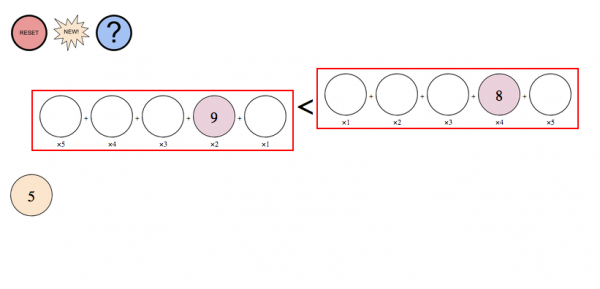Education ∪ Math ∪ TechnologyI created a new puzzle, based on something I played with when I was at the New York Hall of Science. The idea of the puzzle is that given three numbers, you are trying to balance both sides of the equation, with the constraint that different positions on each side of the equation are worth different amounts. At this stage, this is a prototype, so the numbers are chosen randomly, but the eventual plan is to build this balance puzzle into a series of challenges, each of which will probably have multiple solutions.

One hope is that a puzzle like this helps bridge the gap between arithmetic and algebra, by providing an activity which uses arithmetic operations but embeds algebraic reasoning. I also hope that this app helps reinforce that the equal sign (and the inequality symbols) are not indicators that one should do an operation, but rather that they represent a relationship between the two sides of the equation.

Check out the puzzle here: https://davidwees.com/balancepuzzle/

I’d love feedback before I move onto making this puzzle more robust.